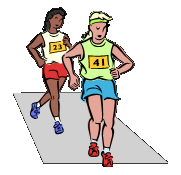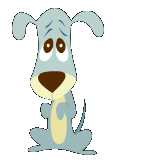# Rounding to the Nearest Whole

You might have seen a word problem that asks you to round to the nearest mile or the nearest dollar. These are examples of rounding to the nearest whole number.

The nearest whole can also be thought of as the ones place. Let's look at our place value chart.Notice that the ones place is to the left of the decimal point.

Let's check out some examples of rounding to the nearest whole.

Example 1: Sheila spent \$156.87.Round this to the nearest dollar.Step 1: Locate the ones place.

\$156.87

Step 2: Use the number to the right to determine if you round up or stay the

\$156.87

The 8 tells us to round up the 6 to a 7.

Step 3: Rewrite the answer ending in the ones place.

\$157

Does our answer make sense? Rounding to the nearest dollar is really just asking, "Is \$156.87 closer to \$156 or \$157? It is only 13 cents away from \$157. So it is definitely closer to \$157. Therefore, this would be our answer when we round.

Example 2: Kyla finished the race in 28.9995seconds. Round to the nearest seconFirst, let's use common sense. Is 28.9995 closer to 28 or 29? We know it is closer to

29 seconds. Now let's show it with our steps.

Step 1:Locate the ones place.

28.9995

Step 2: Use the number to the right to determine if you round up or stay the same.

28.9995

Notice that the number is above 5, so we round up.

Step 3: Rewrite the answer ending in the ones place.

About 29 seconds

Example 3: Fido, the dog weighs 57.221pounds. Round this to the nearest pound.

(Think about it first: Does Fido weight closer to 57 or 58? We can see it is closer to 57! Now let's prove it with the math.)Step 1: Locate the ones place.

57.221

Step 2: Use the tenths place to help round.

57.221

Since the number to the right is less than 5, we leave it

Step 3: Write out the rounded answer.

57 pounds

Example 4:19.831centimeters rounded to the nearest centimeter.(Again, think: is this closer to 19 cm or 20 cm?)

Step 1: Locate the ones place.

19.831

Step 2: Use the tenths place to help round.

19.831

Here, the 8 tells us to round the 9 up to a 10. When this happens the 19 rounds up to 20.

Step 3:< Write out the rounded answer.

20

Let's Review:
When asked to round something to the nearest dollar, minute, second, etc. we are really being asked to round to the nearest ones place. Use the tenths place to help you determine whether the ones place will round up or stay the same. Be sure to drop off all numbers after the decimal point when writing your final answer.

Related Links:
Math
Rounding
Rounding with Decimals
Rounding to the Nearest 10th
Rounding to the Nearest Hundredth
Factors